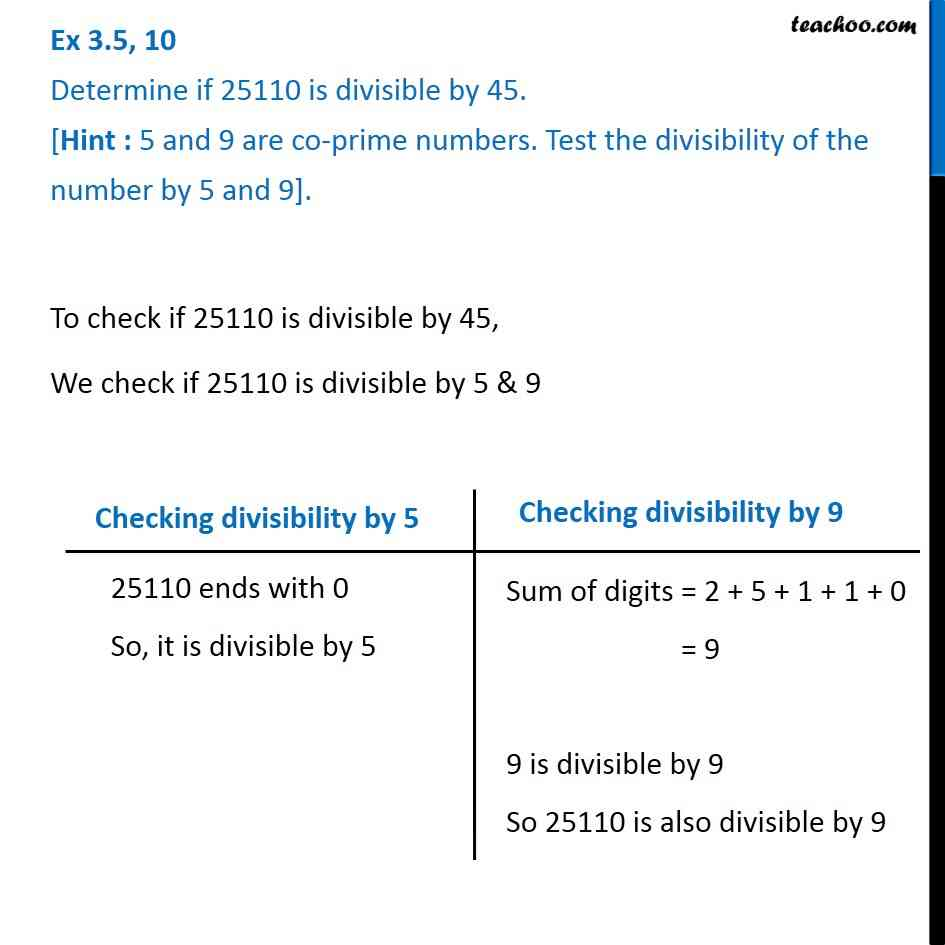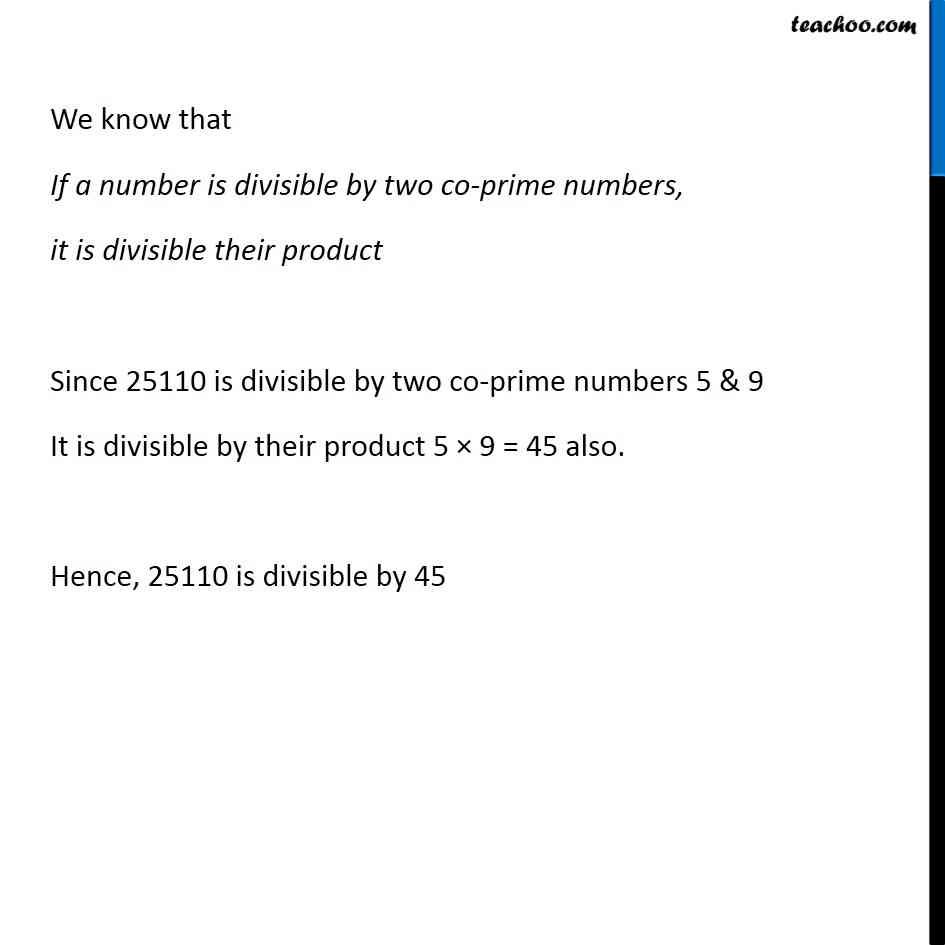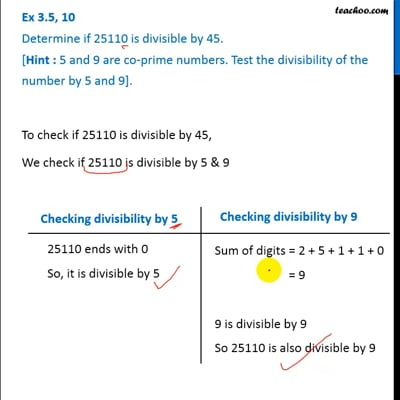Ex 3.5

Chapter 3 Class 6 Playing with Numbers
Serial order wiseThis video is only available for Teachoo black users

Learn in your speed, with individual attention - Teachoo Maths 1-on-1 Class

### Transcript

Question 2 Determine if 25110 is divisible by 45. [Hint : 5 and 9 are co-prime numbers. Test the divisibility of the number by 5 and 9].To check if 25110 is divisible by 45, We check if 25110 is divisible by 5 & 9 Checking divisibility by 5 25110 ends with 0 So, it is divisible by 5 Checking divisibility by 9 Sum of digits = 2 + 5 + 1 + 1 + 0 = 9 9 is divisible by 9 So 25110 is also divisible by 9 We know that If a number is divisible by two co-prime numbers, it is divisible their product Since 25110 is divisible by two co-prime numbers 5 & 9 It is divisible by their product 5 × 9 = 45 also. Hence, 25110 is divisible by 45